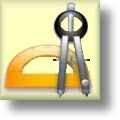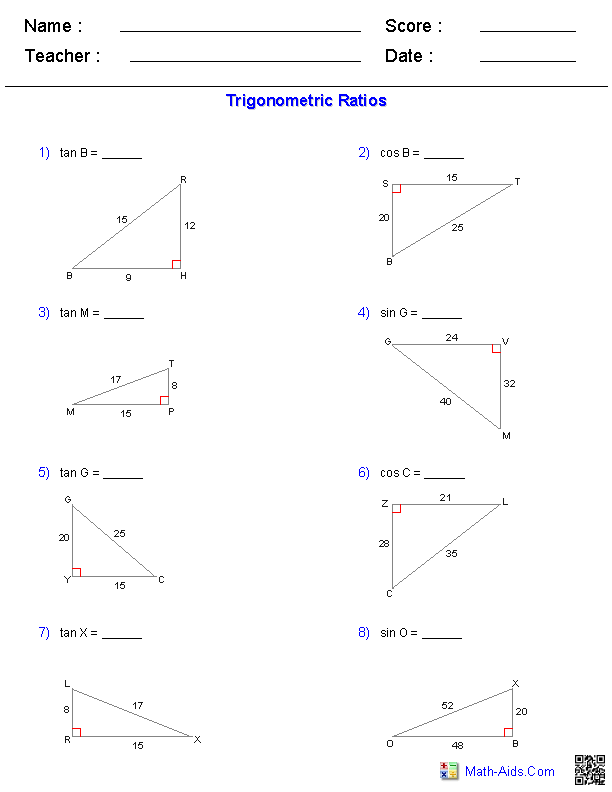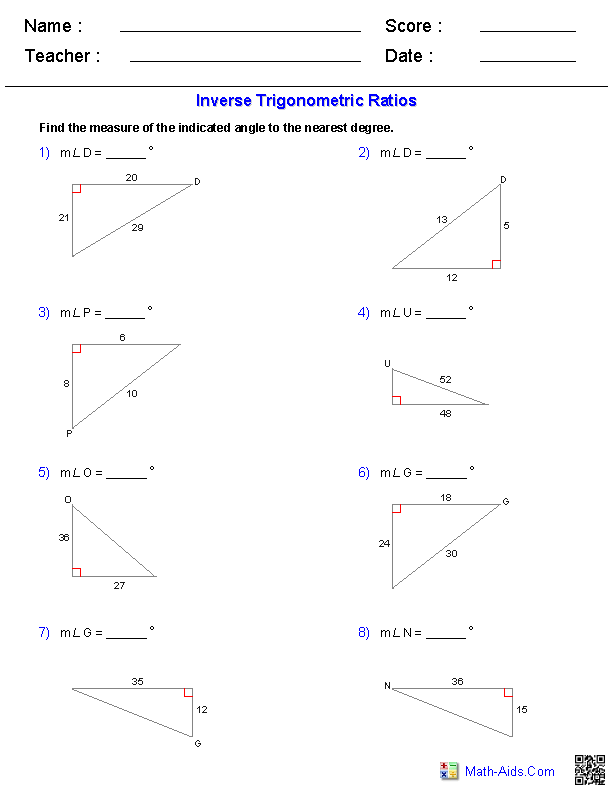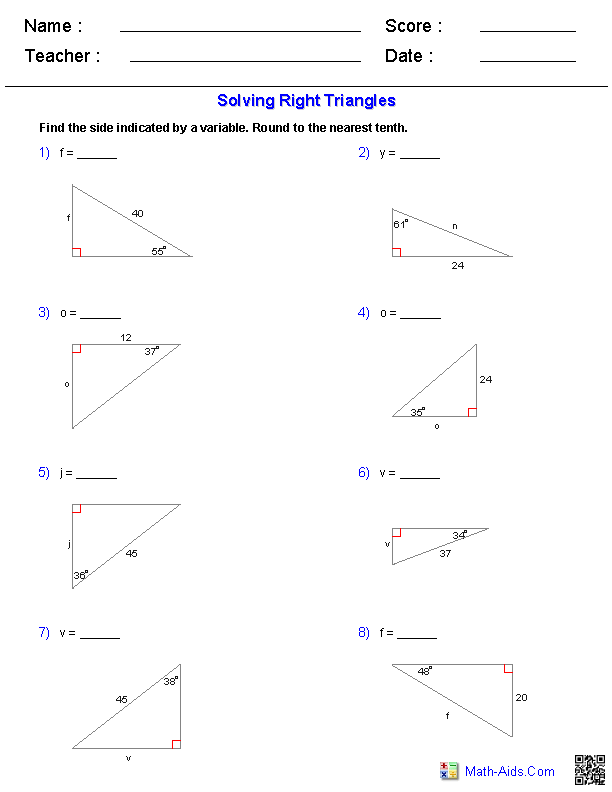Geometry Worksheets

Trigonometry Worksheets

Here is a graphic preview for all of the Trigonometry Worksheets. You can select different variables to customize these Trigonometry Worksheets for your needs. The Trigonometry Worksheets are randomly created and will never repeat so you have an endless supply of quality Trigonometry Worksheets to use in the classroom or at home. We have Trigonometric Ratios, Inverse Trigonometric Ratios, Solving Right Triangles, and Multi-Step Problems Worksheets for your use. Our Trigonometry Worksheets are free to download, easy to use, and very flexible.

These Trigonometry Worksheets are a great resource for children in 5th, 6th Grade, 7th Grade, and 8th Grade.

Quick Link for All Trigonometry Worksheets

Click the image to be taken to that Trigonometry Worksheets.

Trigonometric RatiosTrigonometry WorksheetsInverse Trigonometric RatiosTrigonometry WorksheetsSolving Right TrianglesTrigonometry WorksheetsMulti-Step ProblemsTrigonometry WorksheetsRecommended Videos

Detailed Description for All Trigonometry Worksheets

Trigonometric Ratios Worksheets
This Trigonometry Worksheet will produce trigonometric ratio problems. This worksheet is a great resource for the 5th Grade, 6th Grade, 7th Grade, and 8th Grade.

Inverse Trigonometric Ratios Worksheets
This Trigonometry Worksheet will produce inverse trigonometric ratio problems. You may select the number of sides given for the problems. This worksheet is a great resource for the 5th Grade, 6th Grade, 7th Grade, and 8th Grade.

Solving Right Triangles Worksheets
This Trigonometry Worksheet will produce problems for solving right triangles. This worksheet is a great resource for the 5th Grade, 6th Grade, 7th Grade, and 8th Grade.

Multi-Step Trigomometric Worksheets
This Trigonometry Worksheet will produce multi-step trigonometric problems. This worksheet is a great resource for the 5th Grade, 6th Grade, 7th Grade, and 8th Grade.